# GSEB Solutions Class 10 Maths Chapter 2 Polynomials Ex 2.2

Gujarat Board GSEB Solutions Class 10 Maths Chapter 2 Polynomials Ex 2.2 Textbook Questions and Answers.

## Gujarat Board Textbook Solutions Class 10 Maths Chapter 2 Polynomials Ex 2.2

Question 1.
Find the zeroes of the following polynomial and verify the relationship between the zeroes and the coefficients

1. x2 – 2x – 8
2. 4s2 – 4s + 1
3. 6x3 – 3 – 7x
4. 4u2 + 8u
5. t2 – 15
6. 3x2 – x – 4

Solution:
1. p(x) = x2 – 2x – 8
= x2 – 4x + 2x – 8
[By splitting middle term]
= x(x – 4) + 2(x – 4)
= (x – 4) (x + 2)
For zeroes of p(x),
p(x) = 0
⇒ (x – 4) (x + 2) = 0
⇒ x – 4 = 0
or x + 2 = 0
⇒ x = 4
or x = -2
⇒ x = 4, – 2.
Zeroes of p(x) are 4 and -2.
⇒ α = 4, β = -2Verification of relationship between zeroes and coefficient:
Sum of the zeroes = 4 + (-2) = 2
⇒ α + β = 2
and $$\frac{-b}{a}=\frac{-(\text { coefficient of } x)}{\text { coefficient of } x^{2}}$$
= $$\frac{-(-2)}{1}$$ = 2
⇒ α + β = $$\frac{-b}{a}$$
Product of the zeroes
αβ = 4 × (-2) = -8
and $$\frac{c}{a}=\frac{\text { constant term }}{\text { coefficient of } x^{2}}$$
= $$\frac{-8}{1}$$ = -8
⇒ αβ = $$\frac{c}{a}$$

2. p(s) = 4s2 – 4s + 1
= 4s2 – 2s – 2s + 1
[By splitting middle term]
= 2s(2s – 1) – 1 (2s – 1)
(2s – 1) (2s – 1)
For zeroes of p(s),
P(s) = 0
⇒ (2s – 1) (2s – 1) = 0
⇒ 2s – 1 = 0
or 2s – 1 = 0⇒ s = $$\frac{1}{2}$$
or s = $$\frac{1}{2}$$
⇒ s = $$\frac{1}{2}$$, $$\frac{1}{2}$$
⇒ zeroes of p(s) are $$\frac{1}{2}$$, $$\frac{1}{2}$$
⇒ α = $$\frac{1}{2}$$, β = $$\frac{1}{2}$$
Verification of relationship between zeroes and coefficients:
Sum of the zeroes = $$\frac{1}{2}$$ + $$\frac{1}{2}$$ = 1
⇒ α + β = 1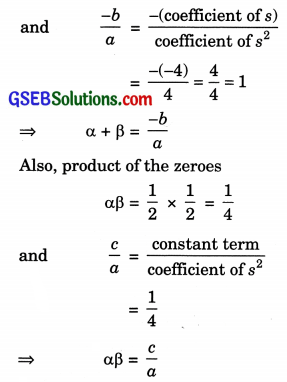3. p(x) = 6x2 – 3 – 7x
p(x) = 6x2 – 7x – 3
= 6x2 – 9x + 2x – 3
= 3x(2x – 3) + 1(2x – 3)
= (2x – 3) (3x + 1)
For zeroes p(x) = 0
⇒ (2x – 3) (3x + 1) = 0
⇒ 2x – 3 = 0
or 3x + 1 = 0⇒ x = $$\frac{3}{2}$$
or x = $$\frac{-1}{3}$$
x = $$\frac{3}{2}$$, $$\frac{-1}{3}$$
⇒ α = $$\frac{3}{2}$$, β = $$\frac{-1}{3}$$
Now, verification of relationship between zeroes and coefficients.
Sum of the zeroes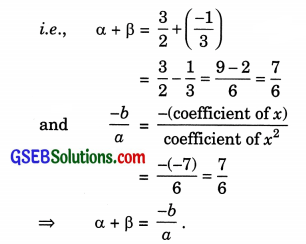Also, product of the zeroes;4. p(u) = 4u2 + 8 u
p(u) = 4u (u + 2)
For zeroes, p(u) = 0
⇒ 4 u(u + 2) = 0
⇒ u(u + 2) = 0
⇒ u = 0
or u + 2 = 0
⇒ u = 0
⇒ u = -2
So, zeroes of p(u) are 0 and -2.
⇒ α = 0, β = -2
Verification of relationship between zeroes and coefficients:
Sum of the zeroes,
α + β = 0 + (-2) = -2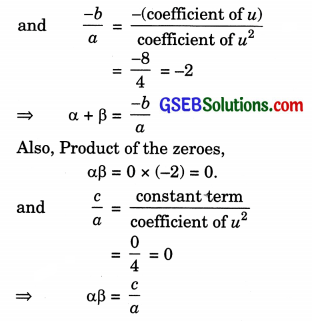5. t2 – 15
P(t) = t2 – 15
For zeroes p(t) = 0
t2 – 15 = 0
t2 – ($$\sqrt { 15 }$$)2 = 0
(t – $$\sqrt { 15 }$$) (t + $$\sqrt { 15 }$$) = o
⇒ t – $$\sqrt { 15 }$$ = 0
or t + $$\sqrt { 15 }$$ = 0
⇒ t = $$\sqrt { 15 }$$
t = –$$\sqrt { 15 }$$
So, zeroes of p(t) are $$\sqrt { 15 }$$ and –$$\sqrt { 15 }$$
⇒ α = $$\sqrt { 15 }$$, β = –$$\sqrt { 15 }$$
Verification of relationship between zeroes
and coefficients:
Sum of the zeroes,
α + β = $$\sqrt { 15 }$$ + (-$$\sqrt { 15 }$$) = 06. p(x) = 3x2 – x – 4
p(x) = 3x2 – 4x + 3x – 4
= x(3x – 4) + 1(3x – 4)
= (3x – 4) (x + 1)
For zeroes, p(x) = 0
⇒ (3x – 4)(x + 1) = 0
⇒ 3x – 4 = 0
or x + 1 = 0
⇒ x = $$\frac{4}{3}$$
or x = -1
⇒ Zeroes of p(x) are $$\frac{4}{3}$$ and -1.
⇒ α = $$\frac{4}{3}$$, β = -1
Verification of relationship between zeroes and coefficients:
Now sum of the zeroes,Question 2.
Find a quadratic polynomial each with the given numbers as the sum and product of its zeroes respectively.

1. $$\frac{1}{4}$$, -1
2. $$\sqrt { 2 }$$, $$\frac{1}{3}$$
3. 0, $$\sqrt { 5 }$$
4. 1, 1
5. $$\frac{-1}{4}$$, $$\frac{1}{4}$$
6. 4, 1

Solution:
1. A quadratic polynomial, when the sum and product of its zeroes are given, is
p(x) = K [x2 – (sum of the zeroes) x + Product of zeroes]
where K is constant.
Now sum of the zeroes = $$\frac{1}{4}$$
Product of the zeroes = -1
∴ Required quadratic polynomial is given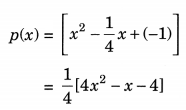or K[4x2 – x – 4]

2. $$\sqrt { 2 }$$, $$\frac{1}{3}$$
Sum of the zeroes, S = $$\sqrt { 2 }$$
Product of the zeroes, P = $$\frac{1}{3}$$
∴ Required polynomial is
p(x) = K[x2 – Sx + P]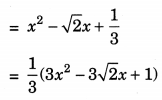3. 0, $$\sqrt { 5 }$$
Sum of the zeroes S = 0
Product of the zeroes, P = $$\sqrt { 5 }$$
∴ Required polynomial is
p(x) = K[x2 – Sx + P]
= x2 – 0x + $$\sqrt { 5 }$$
p(x) = x2 + $$\sqrt { 5 }$$4. 1, 1
Sum of the polynomial, S = 1
Product of the polynomial, P = 1
∴ Required polynomial is
p(x) = K[x2 – Sx + P]
= x2 – 1x + 1
p(x) = x2 – x + 1

5. $$\frac{-1}{4}$$, $$\frac{1}{4}$$
Sum of the zeroes, S = $$\frac{-1}{4}$$
Product of the zeroes, P = $$\frac{1}{4}$$
∴ Required polynomial is
p(x) = K[x2 – Sx + P]6. 4, 1
Sum of the zeroes, S = 4
Product of the zeroes, P = 1
∴ Required polynomial is
p(x) = K[x2 – Sx + P]
p(x) = x2 – 4x + 1